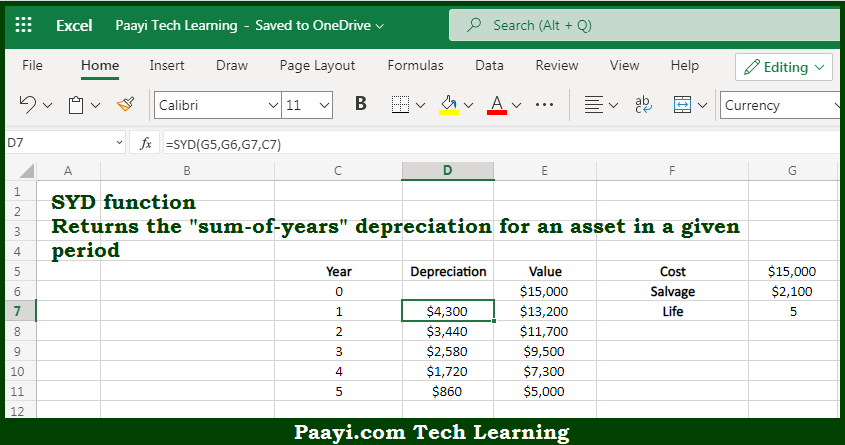# Learn How to Use Microsoft Excel SYD Function

Written by | 0 Comments | 540 Views

In this article, you will learn how to use the Microsoft Excel SYD function and its prime function in Microsoft Excel. You will also get to know the Microsoft Excel SYD function return value and syntax with the help of some examples.

Microsoft Excel SYD Function

The main purpose of the Microsoft Excel SYD function is to get the depreciation for the sum of years. That implies, with the help of the SYD function you can able to return the "sum-of-years" depreciation for an asset in a given period. It should be noted that the calculated depreciation is based on initial salvage value, asset value, and the number of periods on which the asset is getting depreciated. So, with the help of the SYD function, you can able to get the depreciation for the sum of years.

Return Value of SYD Function

The return value will be the depreciation in the given period.

Syntax of SYD Function

=SYD(cost, salvage, life, period)

Where the arguments:

• cost: This is the initial cost of the asset.
• salvage: This is the asset value at the end of depreciation.
• life: This is the period over which the asset is depreciated.
• period: This is the period for which the depreciation is calculated.

How to Use Microsoft Excel SYD Function?So we know that Microsoft Excel SYD function you can able to get the depreciation for the sum of years. That implies, with the help of the SYD function you can able to return the "sum-of-years" depreciation for an asset in a given period. It should be noted that the calculated depreciation is based on initial salvage value, asset value, and the number of periods on which the asset is getting depreciated. So, with the help of the SYD function, you can able to get the depreciation for the sum of years.